# Simulink based Three Phase Voltage Source Inverter simulation

Three Phase Voltage Source Inverter with Simulink Model: The three-phase voltage source inverter is a voltage source inverter that inverts the dc voltages into three phase ac voltages. Normally we have been used two types of inverters with respect to their phases. First one is single phase voltage source inverter which have been used for low power applications and second one is three phase voltage source inverter which have been used for high power applications. The main advantage of these types of inverters is that these are controllable voltage source inverter means the frequency, amplitude and voltage we can easily control. These have been mainly used in UPS (Uninterruptible Power Supply), ASDs (Adjust Speed Drives) and FACTs (Flexible Alternating Current Transmission System) devices. In this article, we will explain how we make a three-phase voltage source inverter in MATLAB Simulink as well we will explain how we make a new model with selection the power electronics components. First, we will open the MATLAB and then click on MATLAB Simulink library then MATLAB Simulink library browser would be opened with a new page shown in figure 1.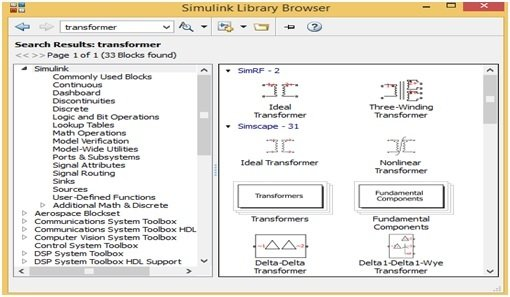Figure 1 MATLAB Simulink Library Browser

This MATLAB Simulink library browser have different options on their Manu bar but for making a new simulating circuit first we must need a new model and then click on new model option then new model would be opened with a new page shown if figure 2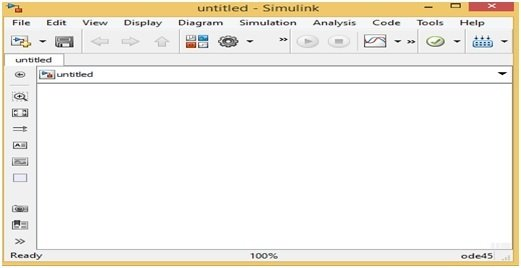Figure 2 MATLAB Simulink New Model

On this MATLAB Simulink new model, we can easily make our new circuits as we want. For making a new circuit first we will choose the power electronics components. For choosing power electronics component, we will just write the word power electronics on search Manu bar of MATLAB Simulink library browser then a new page would be opened which have different blocks of power electronics components shown in figure 3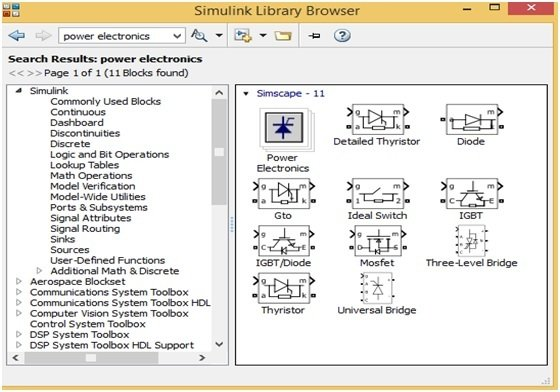Figure 3 Power Electronics Components Blocks

We can easily drag these power electronics blocks on a MATLAB Simulink new model by clicking on any specific block. Without this, we can also add these blocks to new model by just clicking the right click on any block and then click on option Add block to a new model then this block will automatically add to a new Simulink model. Similarly, for choosing the other blocks such as display and oscilloscope just write the word sink on MATLAB Simulink library then a new page would be open shown in figure 4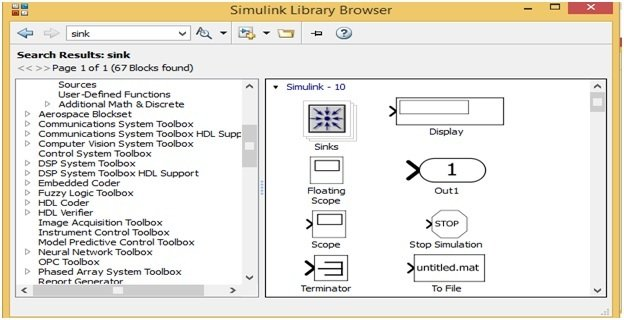Figure 4 Oscilloscope and Display Blocks

Similarly, we can choose and drag all the other blocks such as inductor, capacitor, voltmeter, ammeter, PWM generator and sine wave generator etc. Here we have made a three-phase voltage source inverter with the help of these Simulink blocks which is shown in figure 5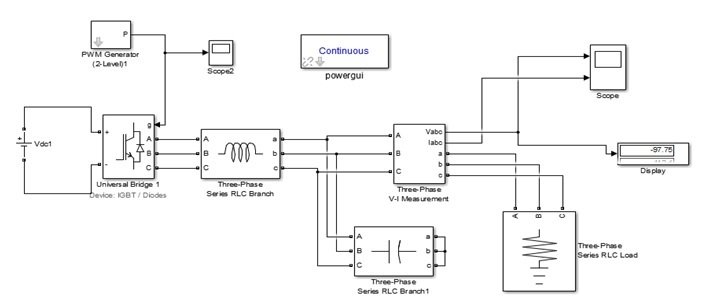Figure 5 Three Phase Voltage Source Inverter with Simulink Model

This three-phase voltage source inverter has made with the help of three phase universal bridge block whose parameters are set according to figure 6 and has powered up with 314V dc.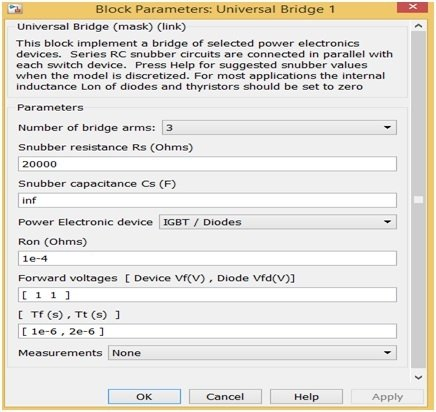Figure 6 Universal Bridge Block Parameters

Tow level PWM generator has been used for giving the pulses of 3 arms of this universal bridge. The parameters are set of this PWM generator block according to figure 7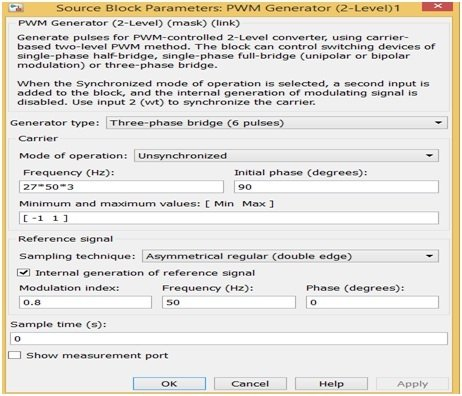Figure 7 PWM Generator Block Parameters

The output of this three-phase voltage source inverter is not pure ac it consists of higher order of harmonic content therefore a LC filter is required for gaining pure ac. Here we have connected a three phase LC filer which have inductance 40 mH and capacitance 120 μF. 1KW load have been used as output load. Its voltage, current waveforms and PWM generator pulses are shown if in figure 8 and 9.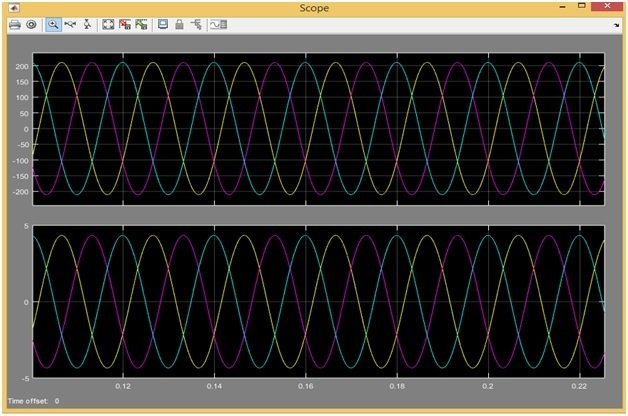Figure 8 Voltage and Current Wave form of Three Phase Voltage Source Inverter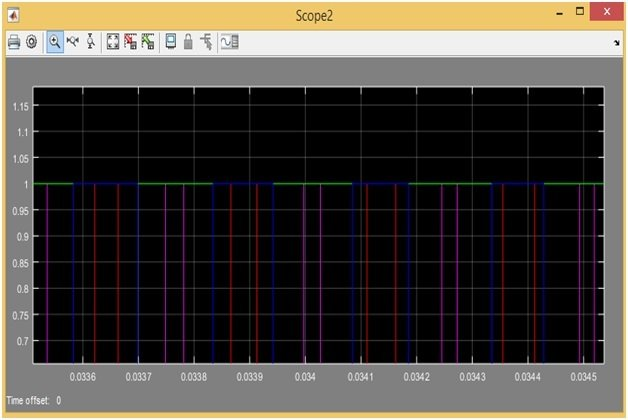Figure 9 PWM Generator Pulses